## Creating a Variance Analysis Model – Part 2

This article builds upon the theory discussed in Creating a Variance Analysis Model – Part 1. Be sure to read this article before looking at the more practical examples below.

## Building a Variance Analysis Model in Excel

Let’s create an Excel model to perform our variance analysis. For the exercise, we will assume a simple scenario for a production company that uses one type of labor, one kind of machine operations, one sort of direct materials and one production process, with one step within it. We will also assume that machine hours for production correspond entirely to labor hours.

### Outline the scenario

To be able to perform our variance analysis, let us start with the following assumptions for one department in the company:

1. The department produces a single product
2. This product is manufactured from a single raw material
3. Direct labor hours for employees and machine working hours are always the same
4. We will perform our analysis in this department only

We need those assumptions to make the model more simplified and facilitate the review. Now let us look at some different types of variances that we can calculate for the business.

### Creating the model

#### Sales Variances

First, we will look at the sales variances for the company. Our input data will be as follows:

• The standard price of the product is 4.40 euro per item
• The actual price we sold it at is 4.50 euro per item
• We sold 18,500 units of the product
• Budgeted quantity at the standard mix of sales was 20,500 units
• The actual quantity sold, applied to the standard mix of sales is equal to 19,000 units

Having set those, we can start calculating the sales variances:

Sales Quantity Variance
= (Actual Quantity at standard mix – Budgeted quantity at standard mix) * Standard price per unit
= (19,000 – 20,500) * 4.40
= -6,600

Sales Mix Variance
= (Actual quantity at actual mix- Actual quantity at standard mix) * Standard price per unit
= (18,500 – 19,000) * 4.40
= -2,200

We combine those two to calculate the Sales Volume variance, which gives us an adverse variance of 8,800 euros.

Moving on to the Sales Price Variance, which shows us the effect of deviations between budgeted and actual price.

Sales Price Variance
= (Actual price – Standard price) * Actual Sold Quantity
= (4.50 – 4.40) * 18,500
= 1,850

As we sold each item of our product at 0.10 euros more than planned, we achieve a favorable sales price variance of 1,850 euros.

Combining the price variance and the quantity variance, we get an overall adverse (negative) Sales Value variance of 6,950 euros.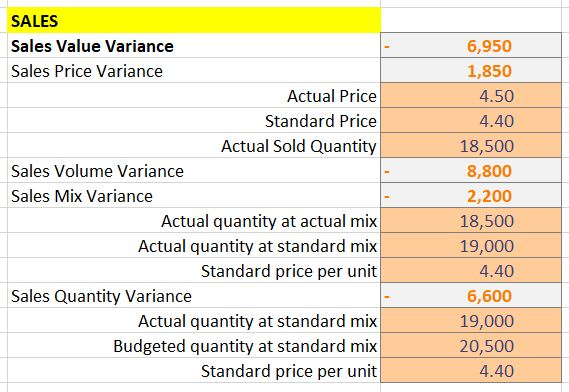This will remain in our calculations sheet, as we will create a more presentable outline in our summary sheet.#### Variable Expenses Variances

Let’s now look at variances we can calculate for the variable expenses.

Starting with direct material expenses, we have the following:

• The actual price of raw material was 2.80 euro per unit
• The standard price is set at 2.70 euro per unit
• The actual quantity used for production was 20,000 units of raw material
• The expected usage of raw material was for 21,000 units

Purchase Price Variance
= (Standard Price – Actual Price) * Actual Quantity
= (2.70 – 2.80) * 20,000
= -2,000

This means that we lost 2,000 euros due to more expensive raw material.

Material Yield Variance
= (Standard Usage – Actual Usage) * Standard price
= (21,000 – 20,000) * 2.70
= 2,700

This is a favorable variance, which shows us we saved 2,700 euros due to using fewer units of raw material than planned.

When we sum the two, we get a favorable Direct Material Variance of 700 euros.And now that we have our calculation, we can include the Direct Material Variance in our summary sheet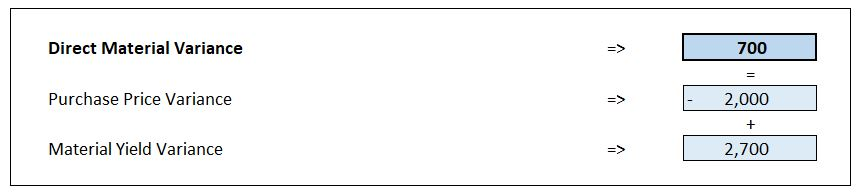After calculating the direct materials variance, we can take a look at the labor expenses. To do that we will need the following information:

• The standard rate per hour is 14.50 euro
• The actual rate at which the company contracted labor was 13.40 euro per hour
• The standard hours for production are 2,300
• The actual hours that production took were 2,500

Direct Labor Price Variance
= (Standard Rate – Actual Rate) * Actual Hours
= (14.50 – 13.40) * 2,500
= 2,750

This is a favorable variance from the fact that the company managed to contract labor at a lower rate.

Direct Labor Quantity Variance
= (Standard Hours – Actual Hours) * Standard Rate
= (2,300 – 2,500) * 14.50
= -2,900

Since it took 200 hours more to complete the work, we have an adverse variance of 2,900 euros.

Combining the two, we arrive at an adverse (negative) total Direct Labor Cost Variance at 150 euros.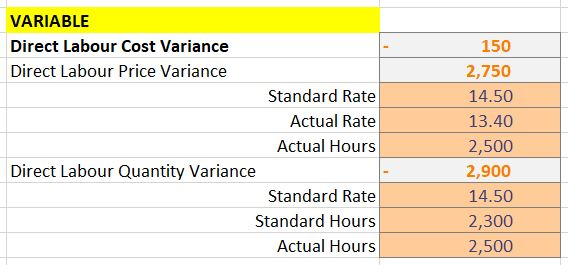After we have our calculation, we can also put the Direct Labor Cost Variance in the summary sheetNext, we can look at the variable production overheads. For this, we will need the following data:

• Actual variable production overheads are at 24,500 euro
• Actual machine hours are 2,500
• Standard machine hours are 2,300
• The Actual overheads absorption rate is 9.80 euro per hour
• The Standard overheads absorption rate is 10 euro per hour

= (Standard Hours – Actual Hours) * Standard Absorption rate
= (2,300 – 2,500) * 10
= -2,000

We get an adverse efficiency variance because the company spent more hours than planned.

= (Actual Hours * Standard Absorption Rate) – Actual Costs Incurred
= (2,500 * 10) – 24,500
= 500

This is a favorable variance since the company incurred lower costs than if valued at the standard absorption rate.

This way, we get an adverse (negative) Variable Production Overhead Variance at 1,500 euros.

We have this in our calculation sheet and put it in the summary sheet as well.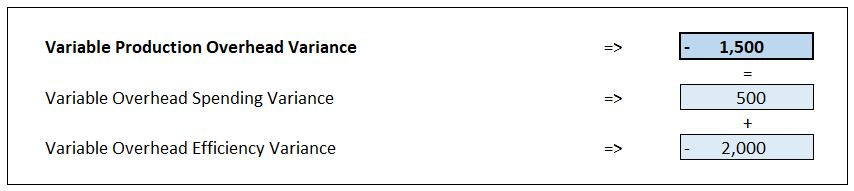• The actual fixed overheads are 15,000 euros
• The budgeted fixed costs were 14,500 euros
• The standard production hours are 2,300
• The budgeted production hours were set at 2,200, pushing for optimization
• The machines worked for 2,500 hours
• The fixed overhead absorption rate is 6 euro per hour

Knowing these details, we can calculate the variances.

= (Standard Production Hours – Actual Production Hours) * Fixed Overhead Absorption Rate
= (2,300 – 2,500) * 6.00
= -1,200

We get an adverse efficiency variance at the amount of 1,200 euros.

= (Budgeted Production Hours – Actual Production Hours) * Fixed Overhead Absorption Rate
= (2,200 – 2,500) * 6.00
= -1,800

The calculation gives a negative value, but this is a favorable variance since the machinery managed to operate for 300 extra hours.

Combining these two, we get a favorable Fixed Overhead Volume variance at the amount of 600 euros.

= 15,000 – 14,500
= 500

We have an adverse (negative) fixed overheads expenditure variance at the amount of 500 euros (the company incurred more fixed overheads than initially budgeted).

Here are our calculations and the entry in the summary sheet.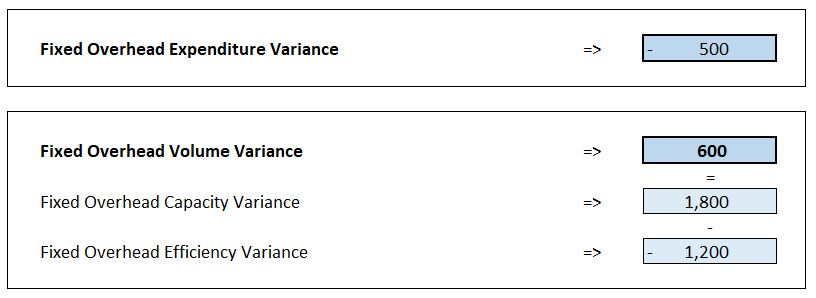## Conclusion

Now we have a more detailed understanding as to how variances analysis works and how to build a simplified model to perform one. After adding some visual representation, we can have a beautiful summary sheet looking like thisThat was the second and last part of Creating a Variance Analysis Model in Excel. You can download the accompanying Excel file below:## FCCA, FMVA

Hi! I am a finance professional with 10+ years of experience in audit, controlling, reporting, financial analysis and modeling. I am excited to delve deep into specifics of various industries, where I can identify the best solutions for clients I work with.

In my spare time, I am into skiing, hiking and running. I am also active on Instagram and YouTube, where I try different ways to express my creative side.